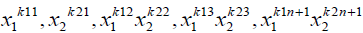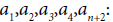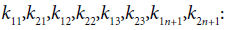#Abstract

The “new square method” is an improved approach based on the “least square method”. It calculates not only the constants and coefficients but also the variables’ power values in a model in the course of data regression calculations, thus bringing about a simpler and more accurate calculation for non-linear data regression processes.

Preface

In non-linear data regression calculations, the “least square method” is applied for mathematical substitutions and transformations in a model, but the regression results may not always be correct, for which we have made improvement on the method adopted and named the improved one as “new square method”.

Principle of New Square Method

While investigating the correlation between variables (x,y), we get a series of paired data (x1,y1,x2,y2……xn,yn) through actual measurements. Plot these data on the x–y coordinates, then a scatter diagram as shown in Figure 1 will be obtained. It can be observed that the points are in the vicinity of a curve, whose fitted equation is set as the following Equation 1.where a0, a1 and k indicate any real numbers.

To establish the fitted equation, the values of a0, a1 and k need to be determined via subtracting the calculated value from the measured value yi, i.e., via (yi–y).

Then calculate the quadratic sum of m (yi–y) as shown in Equation 2.Substitute Expression 1 into Expression 2, as shown in Expression 3:Find the partial derivatives for a0, a1 and k respectively through function Φ so as to make the derivatives equal to zero:Through derivation it is found that there is no analytic solution to this equation set, then computer programs are utilized to calculate its arithmetic solutions and obtain the solutions for a0, a1 and k as well as the correlation coefficient R. It is observed that the closer the correlation coefficient R is to 1, the better the model fits.

Comparison between the “New Square Method” and the “Least Square Method”

If Equation 7 as shown below is adopted to fit any data (Table 1)Table 1: The comparison table between the new square method and the least square method.

• In the “new square method”, the power value k of the dependent variable is calculated, while in the “least square method”, k is assumed to be 1. With the calculated power value for the dependent variable, the “new square method” is able to have the fitted equation generate a fitted line at any curve to better fit the non-linear data .
• In the “new square method”, non-linear data with one factor (x) can be regressed by applying the following Equation 8 in the computer programs to obtain more accurate fittings of non-linear data by regression models .In Equation 8:

• x: Variable;
• y: Function;
• x,y: Dimensional (two-dimensional);
• x^k1,x^k2,x^kn: Element;
• a0: Constant;
• a1,a2,an: Coefficient;
• k1,k2, kn: Power.

As for the regression of non-linear data with multi-factors in the “new square method”, the following Equation 9 can be utilized in computer programs for this purpose. This equation takes into account both the contribution of factors (x1,x2……xn) to the objective function (y) and the interplays among factors (x1,x2……xn) during the regression calculation, that is why the fitted models are of high correlation.In Equation 9:

• x1,x2: Variable;
• y: Function;
• x1,x2,y: Dimensional (three-dimensional);
•: Element;
• a0: Constant;
•Coefficient;
•Power.

Note: Equation 9, which takes three-dimensional data as its example, can be applied for the regression of data in curved surface data.

References

Views: 924

Comment

Join Data Science CentralComment by Peter Blomgren on July 1, 2019 at 9:49am

Mathematicians would call this Nonlinear Least Squares (the model is non-linear in some of the parameters - here the exponents), vs Linear Least Squares (which is linear in ALL the parameters).  A commonly recommended algorithm for solving such problems is the Levenberg-Marquardt Algorithm (see e.g. [1,2,3]).

 "Levenberg–Marquardt algorithm". Wikipedia (https://en.wikipedia.org/wiki/Levenberg%E2%80%93Marquardt_algorithm)

 "Numerical Optimization" Nocedal, Jorge, and Stephen Wright. Springer Science & Business Media, 2006.

 "Numerical Optimization. Lecture Notes 23 - 'Nonlinear Least Squares Problems - Algorithms'". P Blomgren (2018), http://terminus.sdsu.edu/SDSU/Math693a/Lectures/23/lecture.pdfComment by yanping wang on June 26, 2019 at 6:30am

He does not need identity transformation and substitution. There is no analytic solution for the power value, so it can only be solved numerically by computer.Comment by Vincent Granville on June 26, 2019 at 6:21am

How does it compare to linear regression where the variables are transformed using a power transformation, or to polynomial regression?